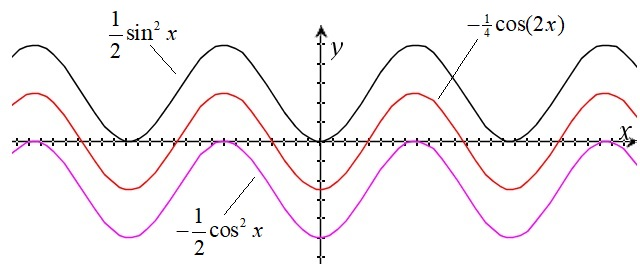# The integral of sin(x)cos(x)dx

Up a level : Integrals
Previous page : One is a logarithm
Next page : The integral of 1/sqrt(1-x^2) dxLet us try to find$\int {\sin x\cos x\;dx}$

in a few different ways

Using a trig rule

We have that$\sin (2\theta ) = 2\sin \theta \cos \theta$

therefore$\begin{gathered} \int {\sin (x)\cos (x)dx} = \int {\tfrac{1}{2}\sin (2x)dx} \hfill \\ \quad = - \tfrac{1}{4}\cos (2x) + C \hfill \\ \end{gathered}$

Here we used the fact that$\int {f(ax + b)dx} = \frac{1}{a}F(ax + b) + C$

The latter can be verified by taking the derivative of the right hand side or using the substitution method with u=ax+b.

Using the substitution u=sin(x)

Let us do a substitution$\left| \begin{gathered} u = \sin (x) \hfill \\ du = \cos x\;dx \hfill \\ \end{gathered} \right.$

So$\begin{gathered} \int {\sin x\cos x\;dx} = \int {udu} \hfill \\ \quad = \frac{{{u^2}}}{2} + C = \frac{1}{2}{\sin ^2}x + C \hfill \\ \end{gathered}$

Using the substitution u=cos(x)

Let’s do the substitution$\left| \begin{gathered} u = \cos (x) \hfill \\ du = - \sin x\;dx \hfill \\ - du = \sin x\;dx \hfill \\ \end{gathered} \right.$

so$\begin{gathered} \int {\sin x\cos x\;dx} = - \int {udu} \hfill \\ \quad = - \frac{{{u^2}}}{2} + C = - \frac{1}{2}{\cos ^2}x + C \hfill \\ \end{gathered}$

Using integration by parts$\begin{gathered} I = \int {\underbrace {\sin x}_g\underbrace {\cos x}_f\;dx} \hfill \\ \quad = \underbrace {\sin x}_F\underbrace {\sin x}_g - \int {\underbrace {\sin x}_F\underbrace {\cos x}_{g'}dx} \hfill \\ \quad = {\sin ^2}(x) - I \hfill \\ \end{gathered}$

So$2I = {\sin ^2}(x) + {C_1}$

Here we added a constant of integration. We finally get$I = \tfrac{1}{2}{\sin ^2}(x) + C$

I.e. the same answer we got with our second method.

Ok, let´s plot the graphs of our three solutions.You will find that they are simply the same solution shifted up or down with some constant.  Given the right values of the respective constant of integration we can make all three solutions overlap.Up a level : Integrals
Previous page : One is a logarithm
Next page : The integral of 1/sqrt(1-x^2) dxLast modified: Jun 19, 2018 @ 16:54